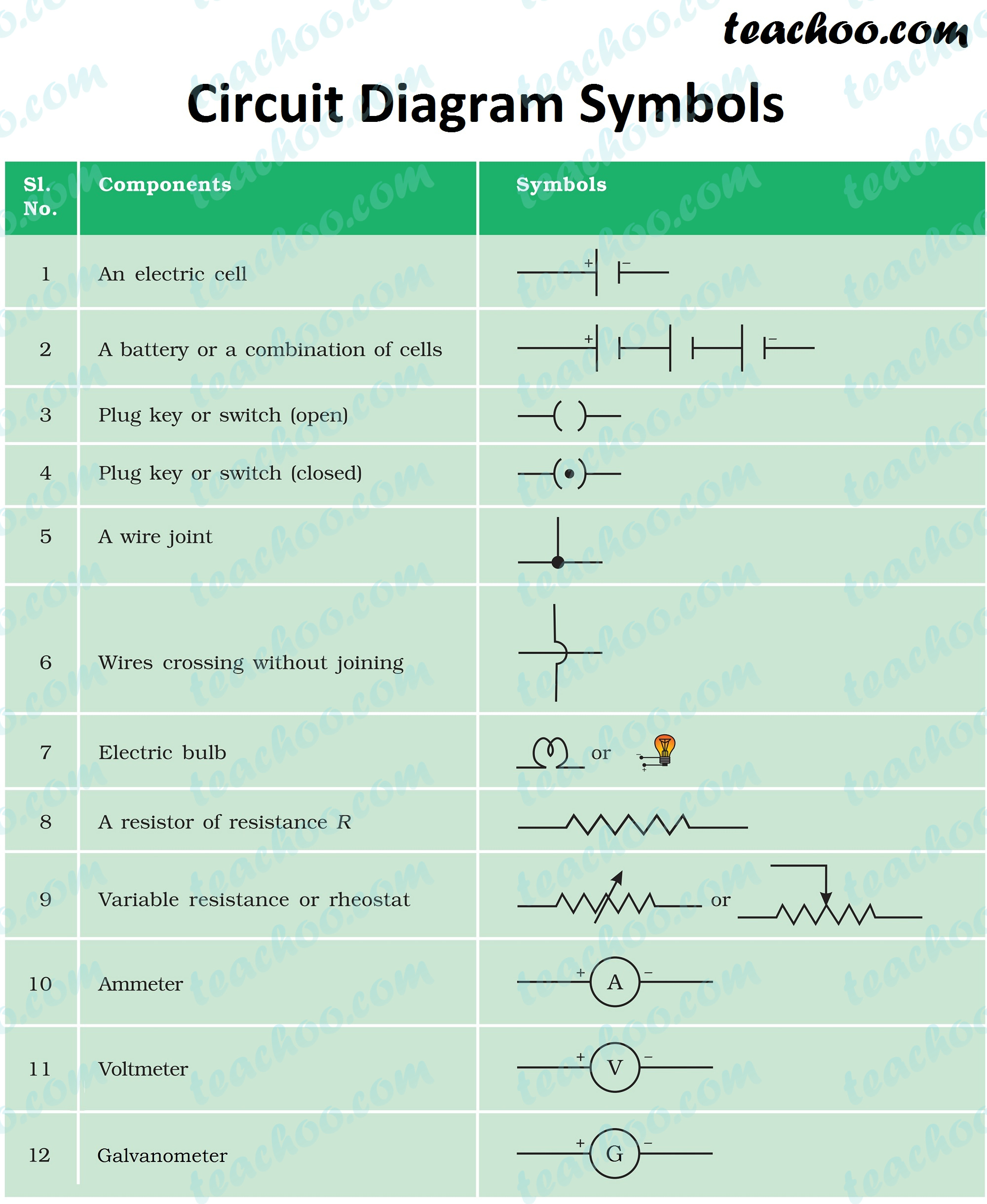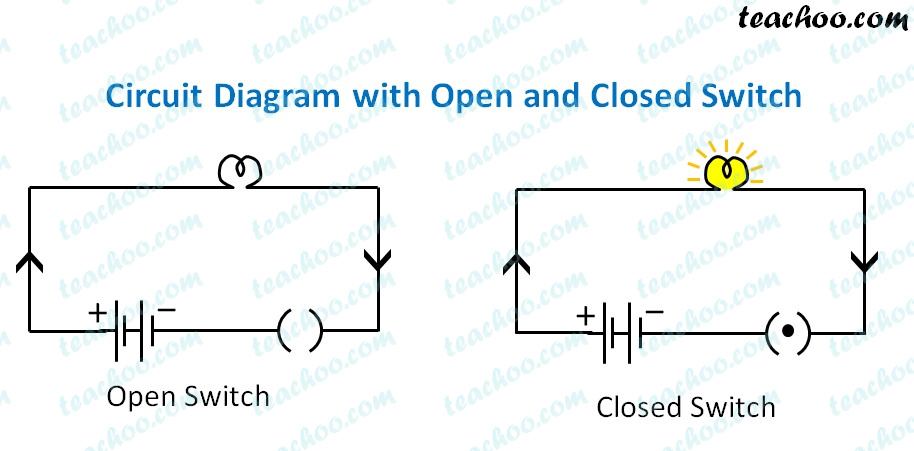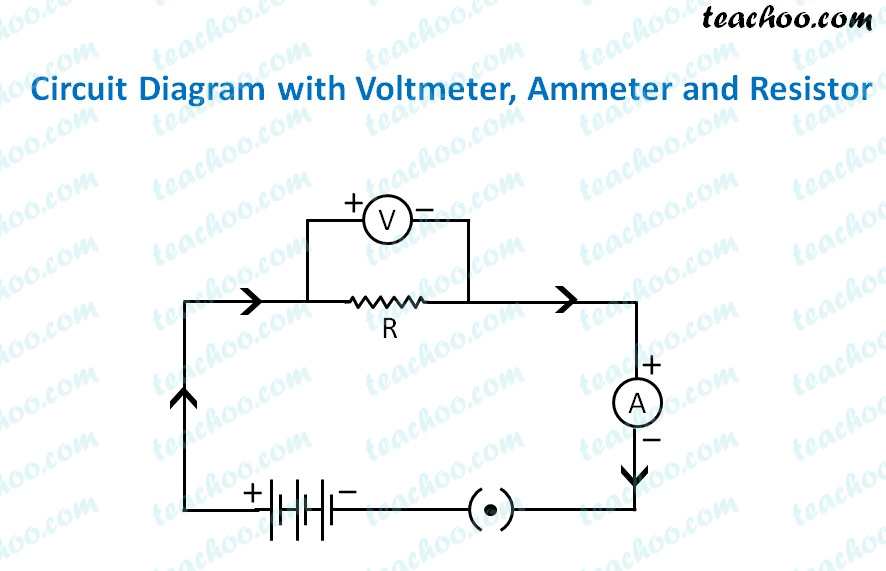Concepts

Class 10
Chapter 12 Class 10 - Electricity

## What is an Electric Circuit

It is a continuous and closed path through which electric current flows

It contains different components like

• Cell or Battery
• Plug Key
• Wires
• Electric Components (like Ammeter,Voltmeter)
• Bulb etc

## What is Circuit Diagram

It is a diagram which shows how different components of circuit are connected

It contains various symbols as shown belowLet's consider a circuit diagram with open and closed switchWhen we close the switch, the bulb glows

Now, let's consider a circuit with Voltmeter, Ammeter and a Resistor
It looks like### Some points to note

• The direction of current is from positive to negative end (though in reality it is the opposite (link)
• The longer end of the battery is the positive end, and the shorter end of the battery is the negative end.
• The positive end of the ammeter is connected to the positive end of the battery, while the negative end of the ammeter is
• connected to the negative end of the battery.
• The positive end of the voltmeter is connected to the positive end of the battery, while the negative end of the voltmeter is
connected to the negative end of the battery.

### Ammeter

Ammeter is the device used to measure the electric current in a circuit.

### Voltmeter

Voltmeter is the device used to measure the potential difference/voltage in a circuit.

A voltmeter is connected across a resistor or a combination of resistors.

### Rheostat

The component used to regulate current without changing the voltage is called variable resistance or rheostat.

## Electric Current is flow of electrons(negatively charged particles)

Electric Current moves from negative Side of battery to positive Side of battery

## How does Electric Current Flow in a Circuit Diagram

In Circuit Diagram, it is represented that

Electric Current flows From Positive end to negative end

This is because when electricity was first discovered,the existence of electrons was not known

However, in reality, it is the opposite.

Electric Current flows from Negative end of battery to positive end

## Questions

Q1 Page 200 - What does an electric circuit mean?

Extra Question 10 - A child has drawn the electric circuit to study Ohm’s law as shown in Figure 12.6. His teacher told that the circuit diagram needs correction. Study the circuit diagram and redraw it after making all corrections.

Extra Question 12 - Draw a circuit diagram of an electric circuit containing a cell, a key, an ammeter, a resistor of 2 Ω in series with a combination of two resistors (4 Ω each) in parallel and a voltmeter across the parallel combination. Will the potential difference across the 2 Ω resistor be the same as that across the parallel combination of 4Ω resistors? Give reason.

Learn in your speed, with individual attention - Teachoo Maths 1-on-1 Class